Like   Pin

## Thursday, October 3, 2019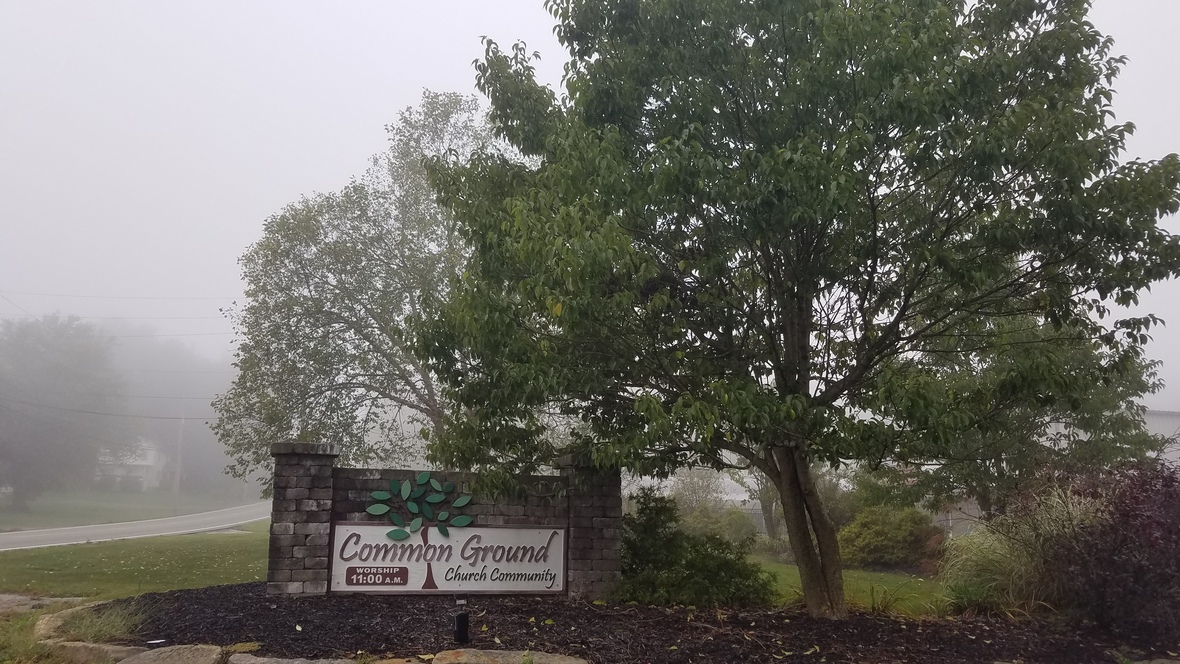table div table+table+table+table div table{width:100%;padding:0}table div table+table+table+table div table img{width:96.23%;padding:0;float:none}table div table+table+table+table div table td{width:100%;padding:0 1.88% 18px}/* styles */# Sunday's Service - October 6, 2019

 /* styles */ We welcome Pastor David Brown to lead us in Worship this week. David is a church planter from the Evangelical Covenant Church. He lives in Columbiana with his wife, Brandi, and their daughter. The featured Scripture comes from: Ephesians 3:14-21 for David's message: Moving In: A Prayer for Power.
 /* styles */ ~~~

# CG NEWS & EVENTS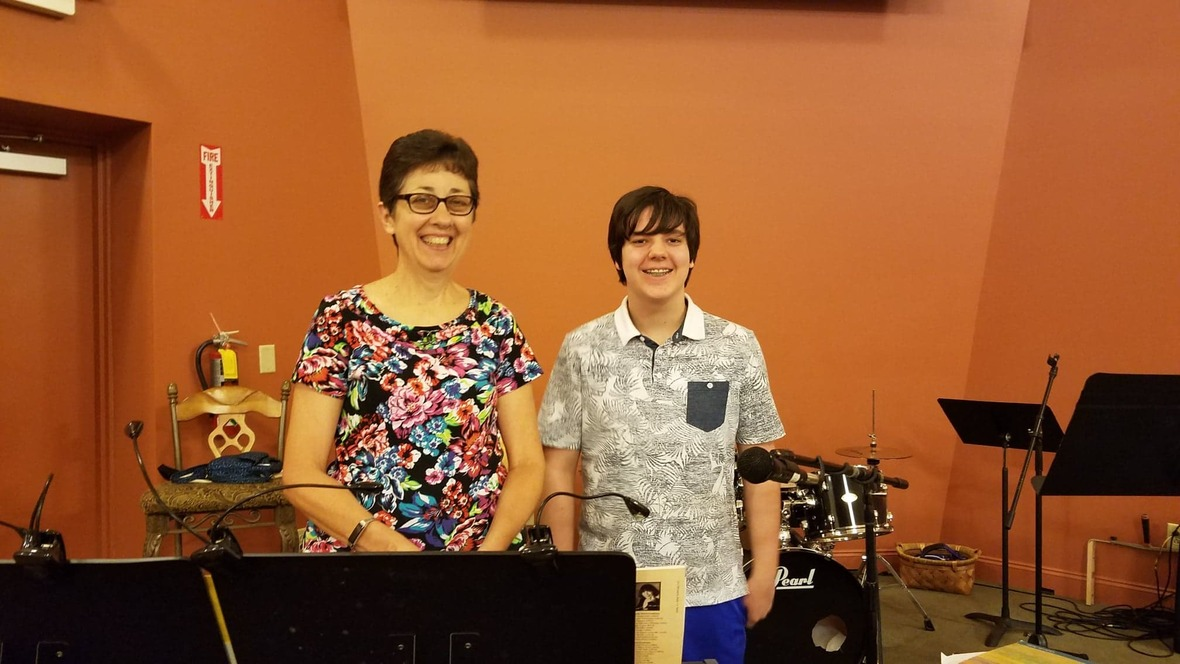## Special Happenings ~ This Sunday

We are excited about worshiping with you on Sunday. Our music leaders will be Janine Landis and Jack Snyder, teaming up for the very first time! Janine will be on the keyboard and the younger Jack Snyder will provide lead vocals to guide us through all the worship songs. (Thanks Jack and Janine!) Additionally, Special Music will also be something new. Brianna Windon will be bringing her dance team from Youngstown Christian School, including Isabella Henceroth, to perform for us! (Thanks Brianna and Izzy!) We hope everyone will join us in this special day of praising the Lord.

~~~~## Bible Studies

Sunday Morning Class: Romans, 9:30a.m.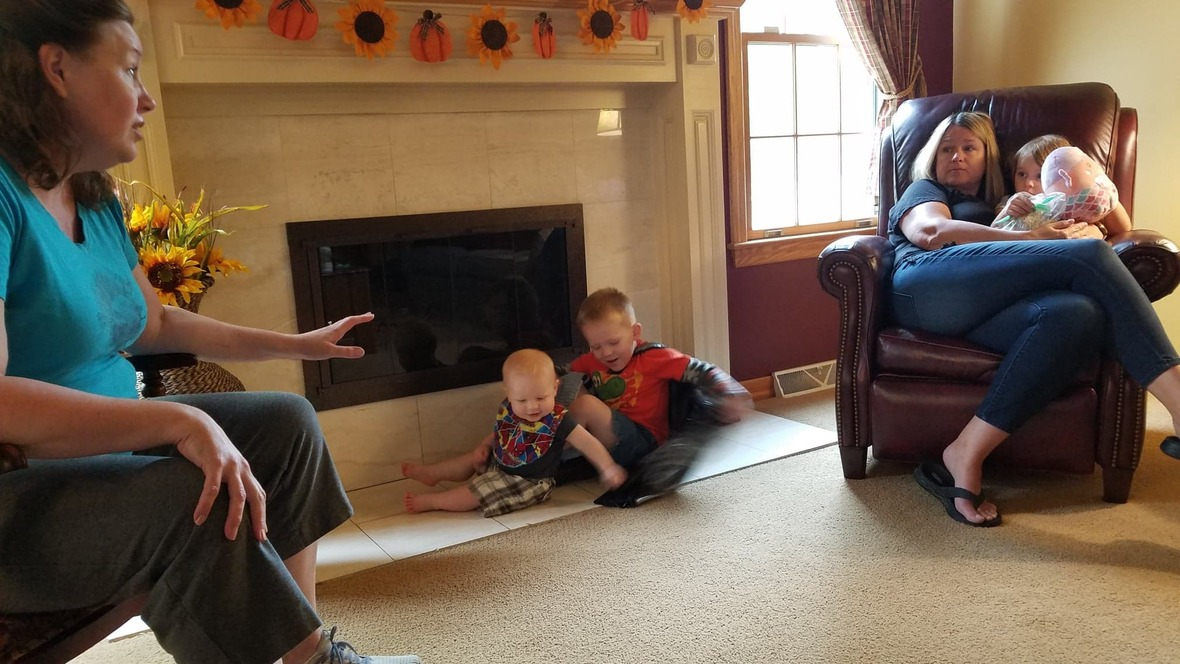Wednesday's Bible Study

Best Friends with God: Falling in Love with the God Who Loves You by Christy Bower.

You are welcome to join in studies at any time!

## Garden & Nursery News

Garden kids will remain in Worship to enjoy this musical service. Activity pages for kids on the table by Sanctuary doors. Children 3 & younger are invited to the Nursery for Bible story time.

Want to volunteer in the Garden and/or with the Sprouts and Nursery age kids?
We would love to have you jump on board! Talk to Jen L. for more information.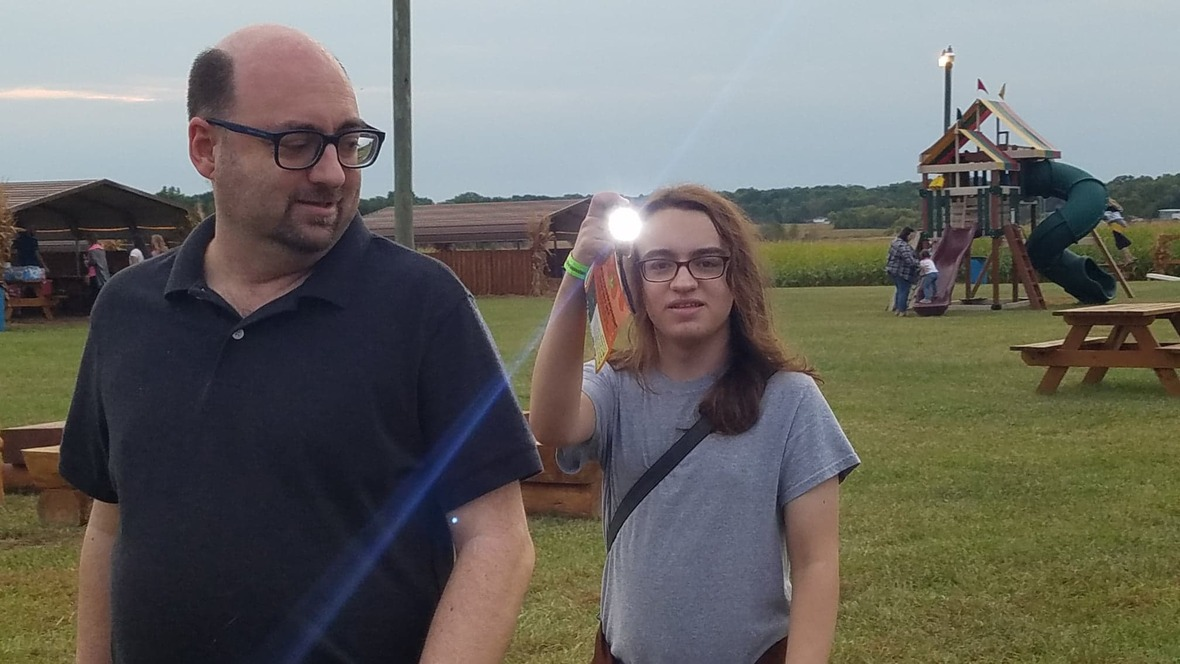## Youth Group

For youth in 5th grade & up

Last Sunday we had a great trip to the Maze Craze. Thank you to everyone who helped make this annual tradition so much fun!

This Sunday we will be focusing on Service. We will be making cards for Aunt Elaine, as she battles cancer, as well as working on getting set up for the Coat & Clothing (& More!) Giveaway.

We invite all the youth to join us for fun, fellowship, and learning this year,
most Sunday evenings from 6-7:30pm.

Snack this week: Chris Lynch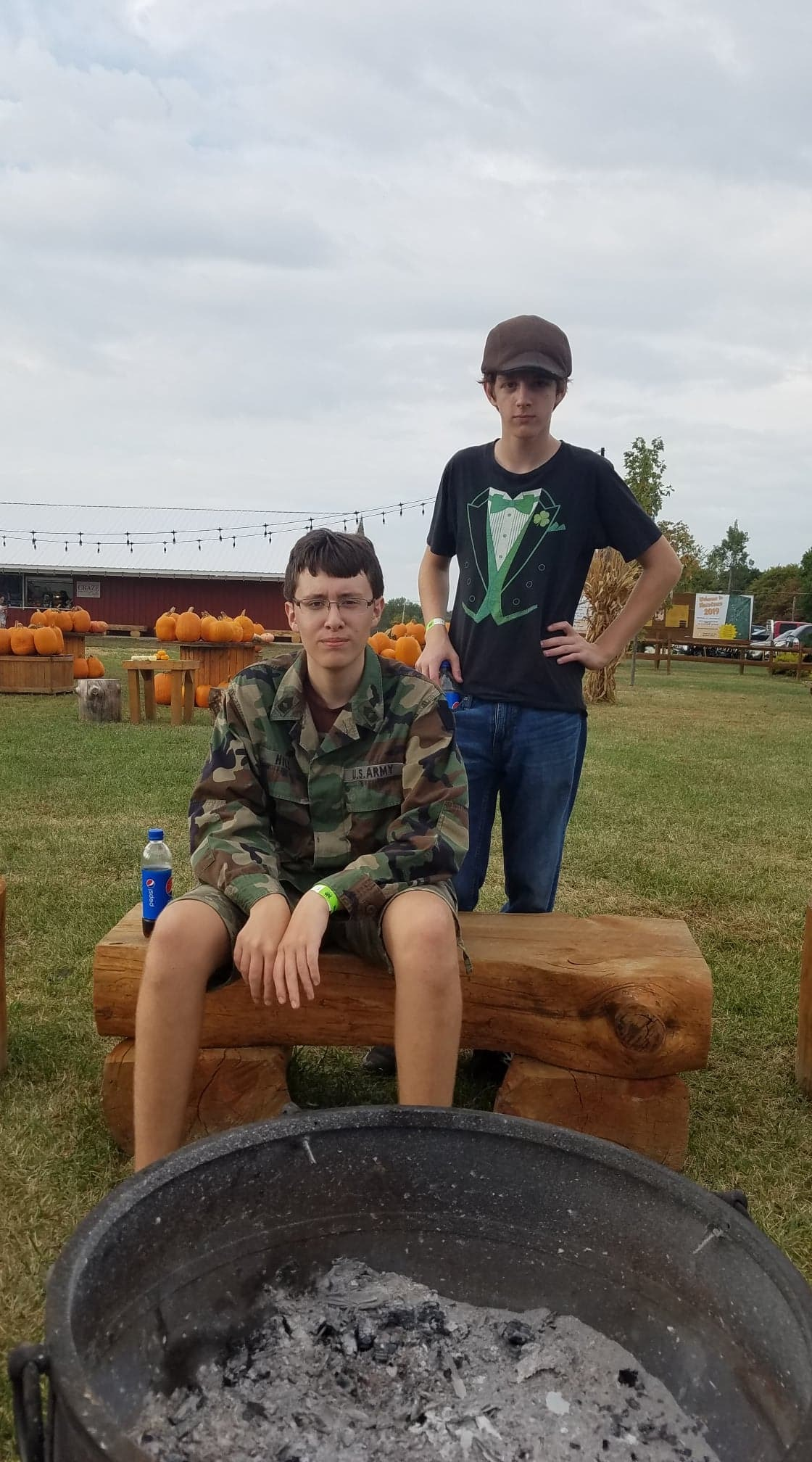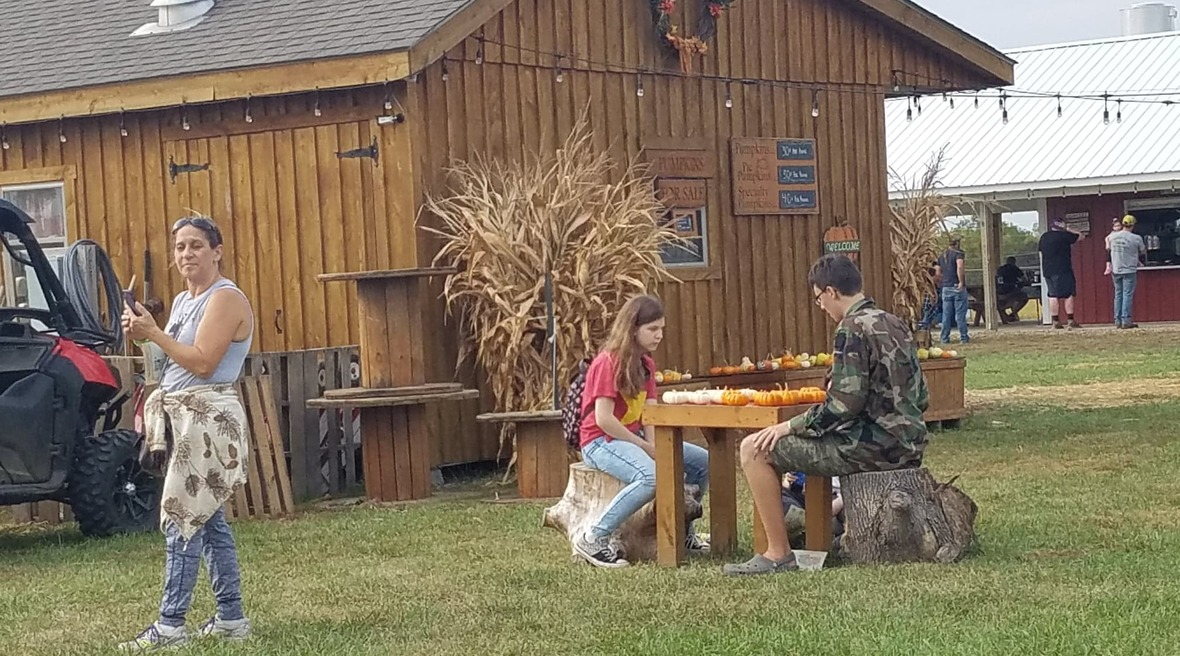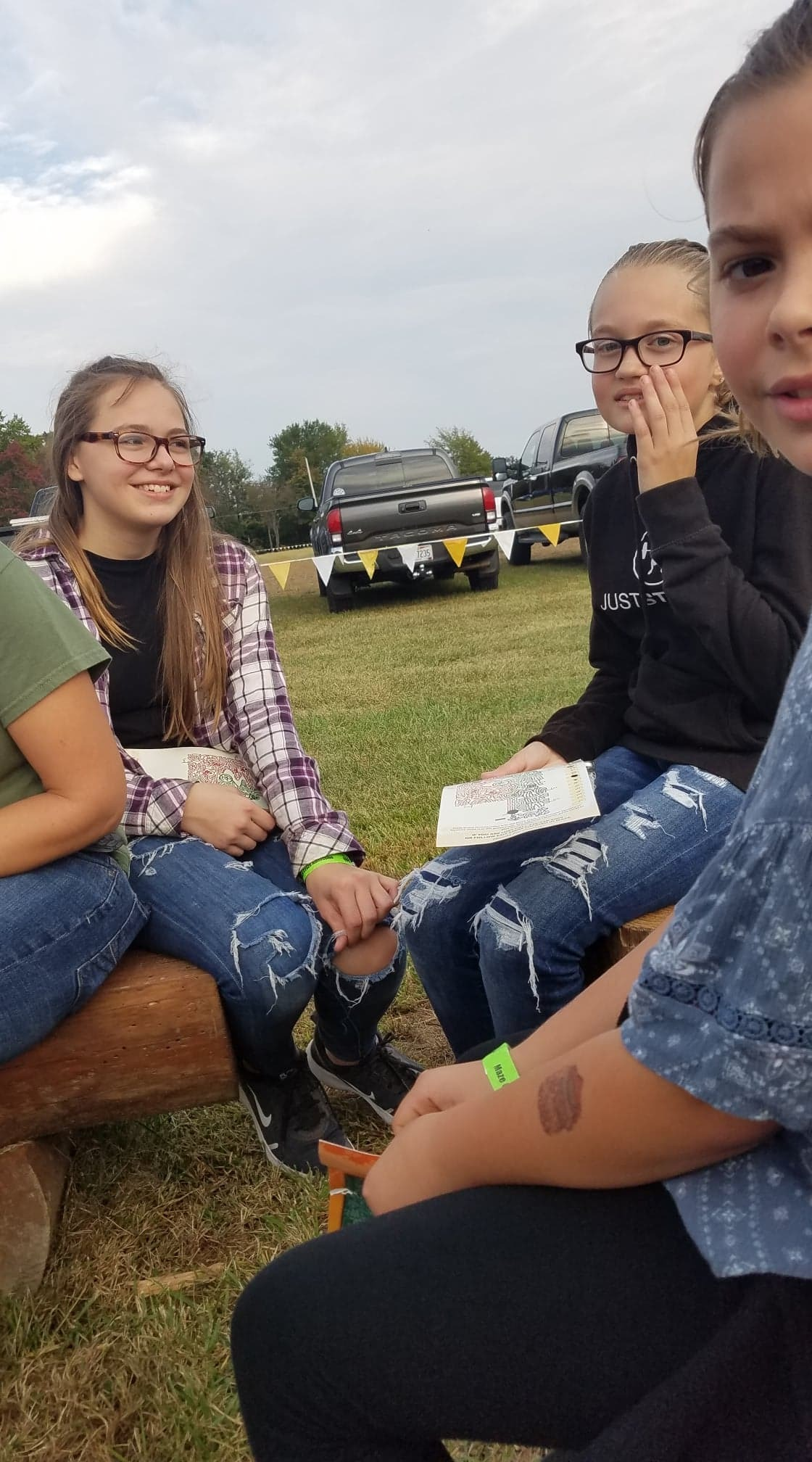/* styles */ At the Maze Craze!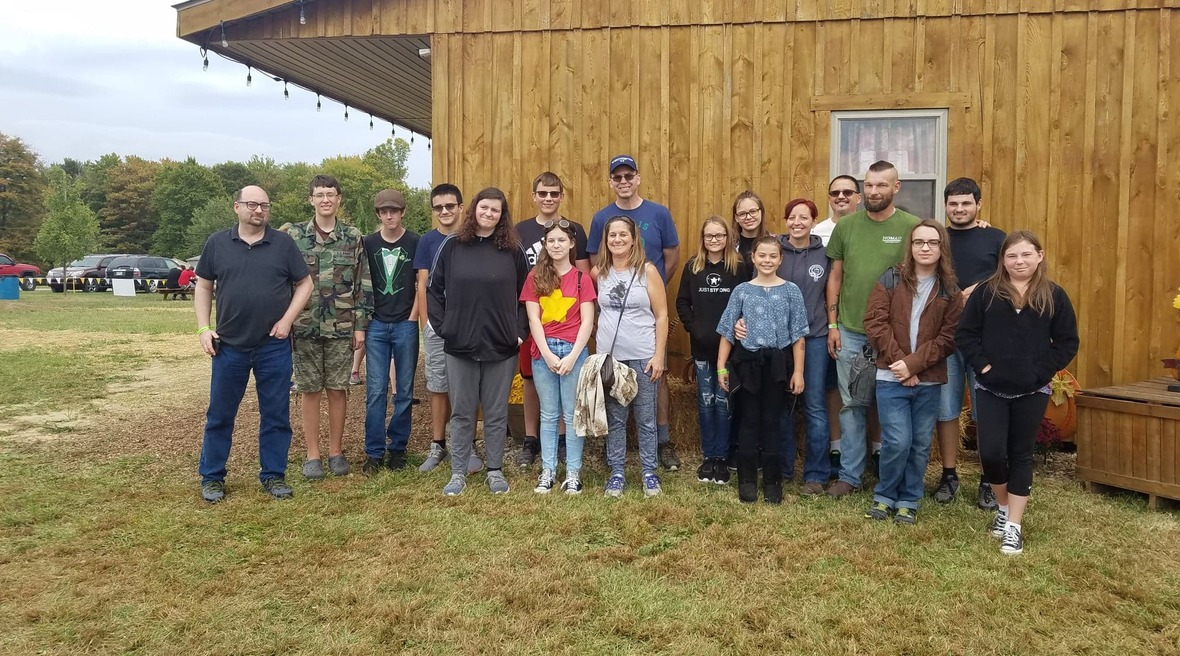table div table+table+table+table+table+table+table+table+table+table+table+table+table+table+table+table+table+table div table{width:100%;padding:0}table div table+table+table+table+table+table+table+table+table+table+table+table+table+table+table+table+table+table div table img{width:96.23%;padding:0;float:none}table div table+table+table+table+table+table+table+table+table+table+table+table+table+table+table+table+table+table div table td{width:100%;padding:0 1.88% 18px}/* styles */# UPCOMING AT CG## October Speakers

13th - Phil Bartholomew
20th - Adam Shank - Communion Sunday
27th - John Muckridge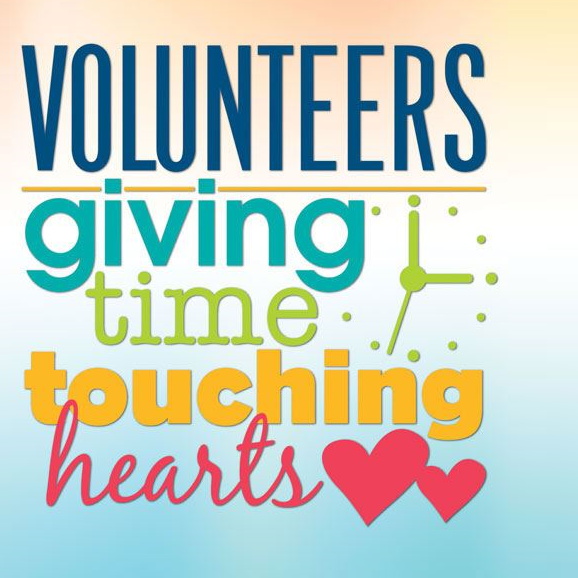## YG Service Opportunity at Our Giveaway

Saturday, October 12 from 9:30am – 1:30pm
Many hands make light work! We can use all hands on deck before, during, and after our Coat & Clothing Giveaway.

Youth Group will not meet on Sunday evening the weekend of the Giveaway, so youth are encouraged to spend some time with us on that Saturday, helping to serve the community.

“Whoever brings blessing will be enriched, and one who waters will himself be watered.”
Proverbs 11:25## Saturday, October 19 from 2-4pm

Are you ready to gather and celebrate the fall harvest, like we do each October? This year we are adding a little twist! On this exact date in 2008 (11 years ago!), CGCC held our first service in our building! Since it is our 11th "birthday" we felt it would be a great time to celebrate. So, we will continue our annual fall tradition and add in some birthday fun!What will you carve?

We will also be having a pumpkin carving contest. All ages are invited to carve a jack o'lantern (or two) and bring them here for judging and fun.

Keep an eye out for further details
to be announced soon.

 table div table+table+table+table+table+table+table+table+table+table+table+table+table+table+table+table+table+table+table+table+table+table+table+table div table{width:100%;padding:0}table div table+table+table+table+table+table+table+table+table+table+table+table+table+table+table+table+table+table+table+table+table+table+table+table div table img{width:96.23%;padding:0;float:none}table div table+table+table+table+table+table+table+table+table+table+table+table+table+table+table+table+table+table+table+table+table+table+table+table div table td{width:100%;padding:0 1.88% 18px}/* styles *//* styles */ www.goodnessgrows4all.org Our Mission Statement: To cultivate personal, social, and community growth through gardening, education, and opportunity for people of all abilities. ~~~

# NEWS & EVENTS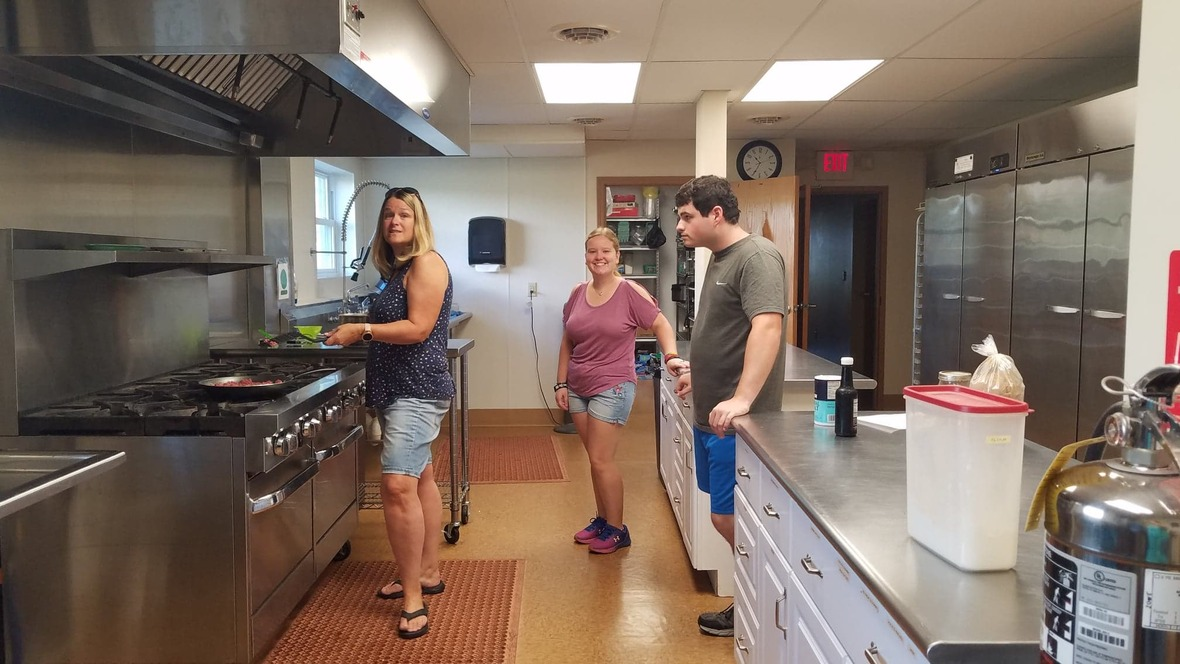## Fall at GG

Lots of prep work going on here for October 12th's Giveaway. We have been busy sorting, folding, and stacking clothes as well as moving tables. Our school friends have also been a big help with this!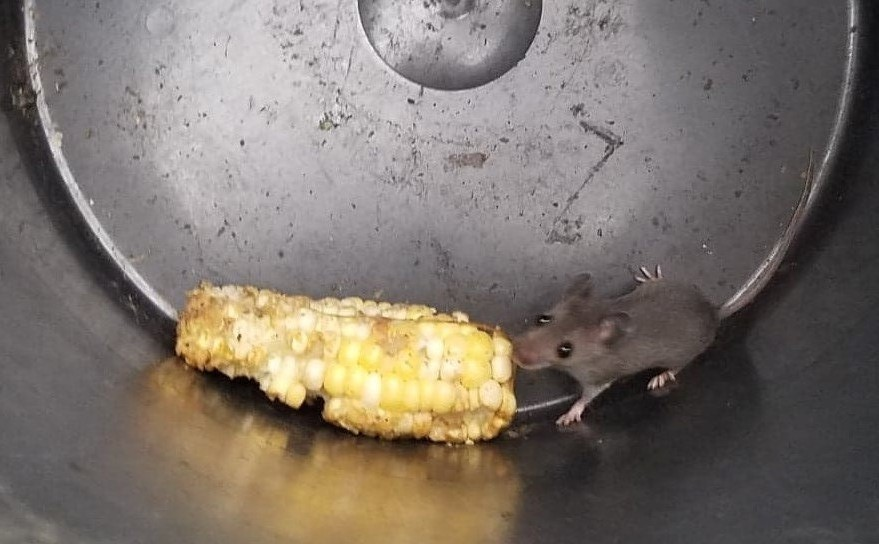Greenhouse Mouse!

Tuesday's lunch was the best sloppy joe sandwich you could ever imagine! Miss Jill was our head cook, leading Tori and Dustin in following Rachel's Grandma Long's recipe. They paired that with fries, apple slices, and fresh green beans. It was another day of good eating at GG!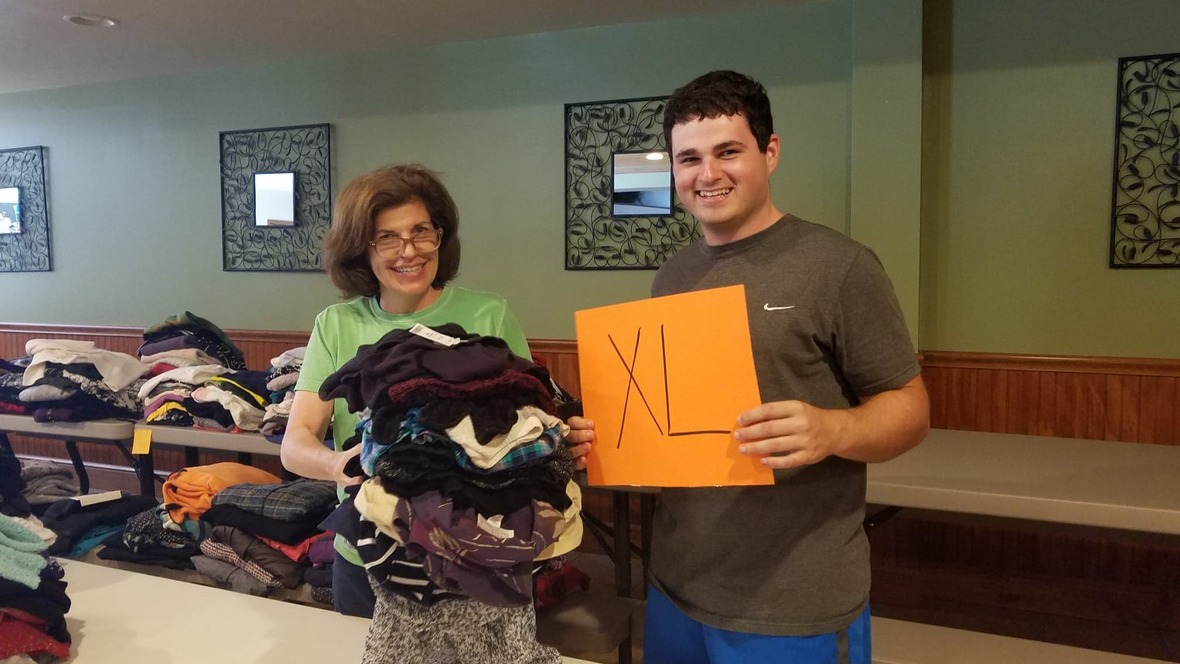Today is CSA day. Only one more week left! This week our crew made fresh apple bread (from apples Keegan picked at an orchard) and homemade salsa verde. It is an exciting share day!

Miss Barb came for a working visit on Tuesday. She dove in and helped with the clothing sorting. Thanks, Miss Barb! We enjoyed having you here! See you next week!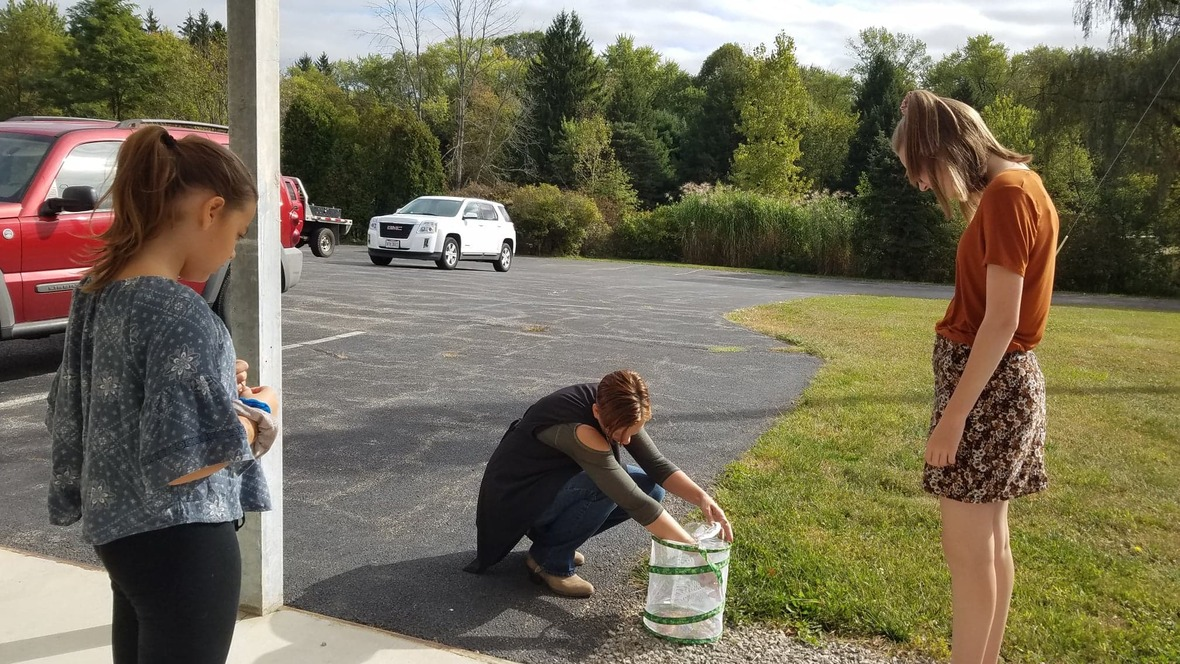This week's butterfly release.
 table div table+table+table+table+table+table+table+table+table+table+table+table+table+table+table+table+table+table+table+table+table+table+table+table+table+table+table+table+table+table+table+table div table{width:100%;padding:0}table div table+table+table+table+table+table+table+table+table+table+table+table+table+table+table+table+table+table+table+table+table+table+table+table+table+table+table+table+table+table+table+table div table img{width:96.23%;padding:0;float:none}table div table+table+table+table+table+table+table+table+table+table+table+table+table+table+table+table+table+table+table+table+table+table+table+table+table+table+table+table+table+table+table+table div table td{width:100%;padding:0 1.88% 18px}/* styles */# OUTREACH and MISSION OPPORTUNITIES## Coat & Clothing Giveaway

Saturday, October 12, 10am-1pm
Getting down to the wire here for donations! If you have anything you want to clear out to pass onto other folks, now is the time. No need to even carry donations upstairs now. Please leave items in the donation barrel in the Great Room or bring them to the front desk.

Let your friends and family know that we are accepting donations and that anyone is free to come "shop" with us on October 12th! No residency or proof of income requirements. Help us spread the word!

We are happy to take coats, clothes, shoes, purses and bags, jewelry, hats, gloves and mittens, scarves, and even small household items. We will accept anything that is clean and in good repair that you think someone else might be able to use! Last year, items like purses, shoes, and baby clothes were in high demand. Folks also enjoyed the random item table that had pictures, blankets, glasses, etc. You never know what might make someone's day. One man's trash is another man's treasure!

 /* styles */ Here is the link on Facebook: Giveaway Event Here is the link on allevents.in website: Coat & Clothing Giveaway Please share this event with everyone you know. Some folks may have items to donate, some folks may want to attend, some may want to do both, and we would love to reach them all! Text, call, or email all your friends and family!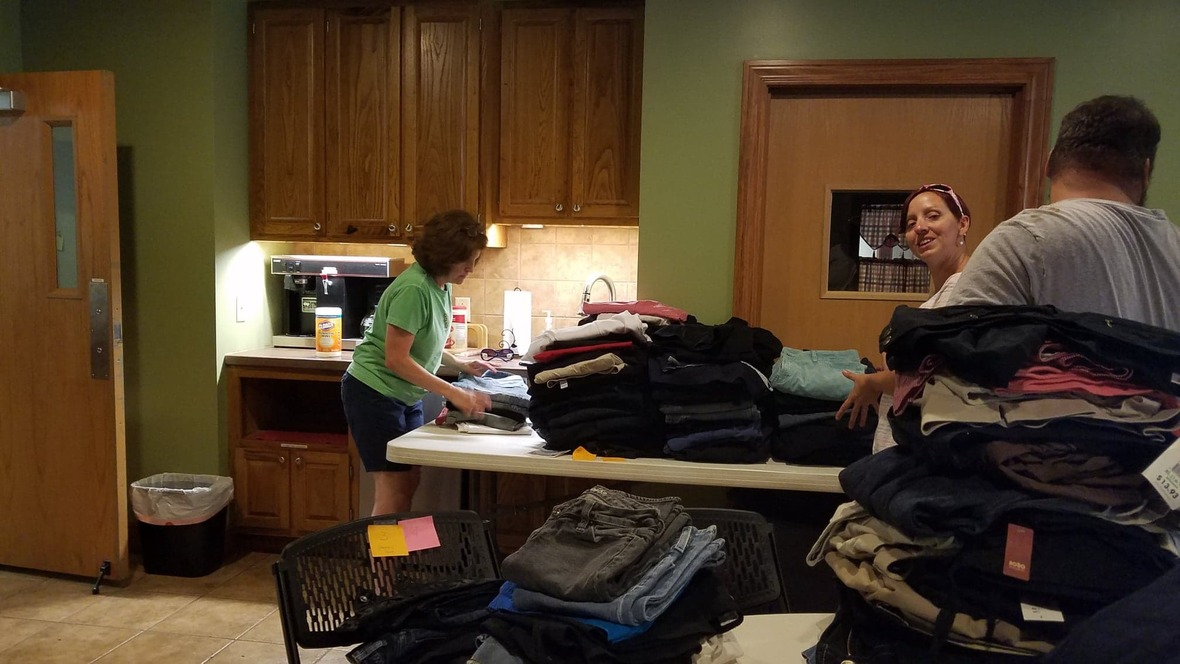## Giveaway Volunteers Appreciated!

We have begun the process of getting ready for the third annual Coat & Clothing Giveaway. There is much to be done in the way of sorting, organizing, and setting up. If you have some time to spare, let us know. All help is appreciated, whether in these set up days, the day of the event, or in the days after for cleanup.

Do you have clothing racks we could borrow? They would definitely come in handy!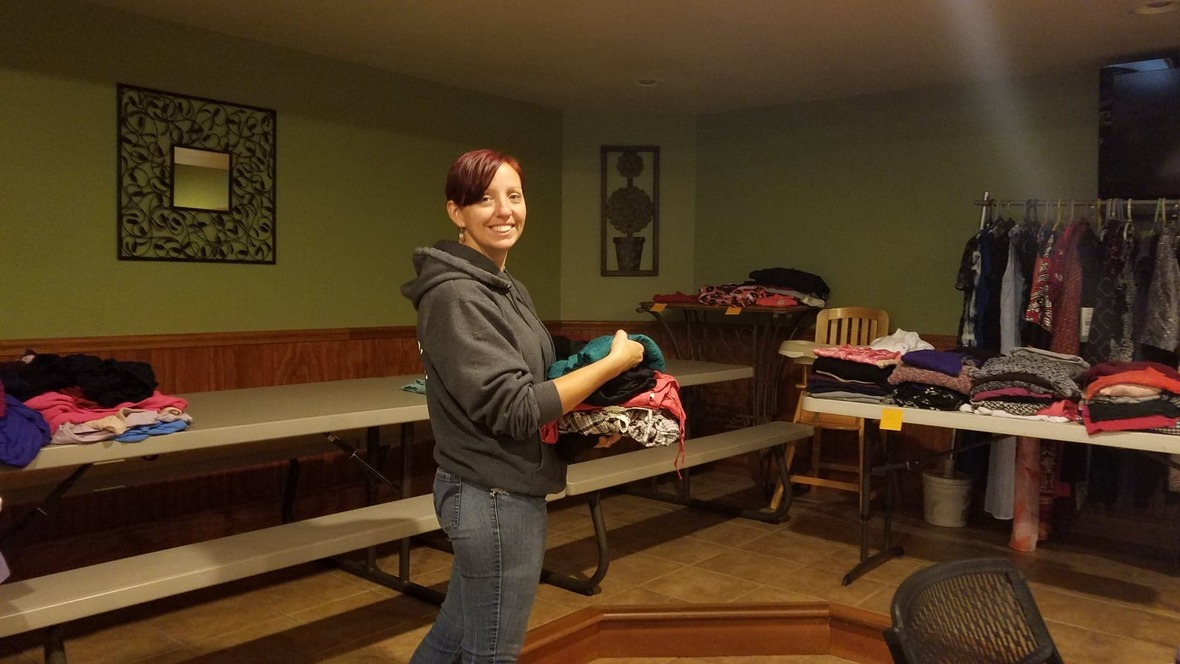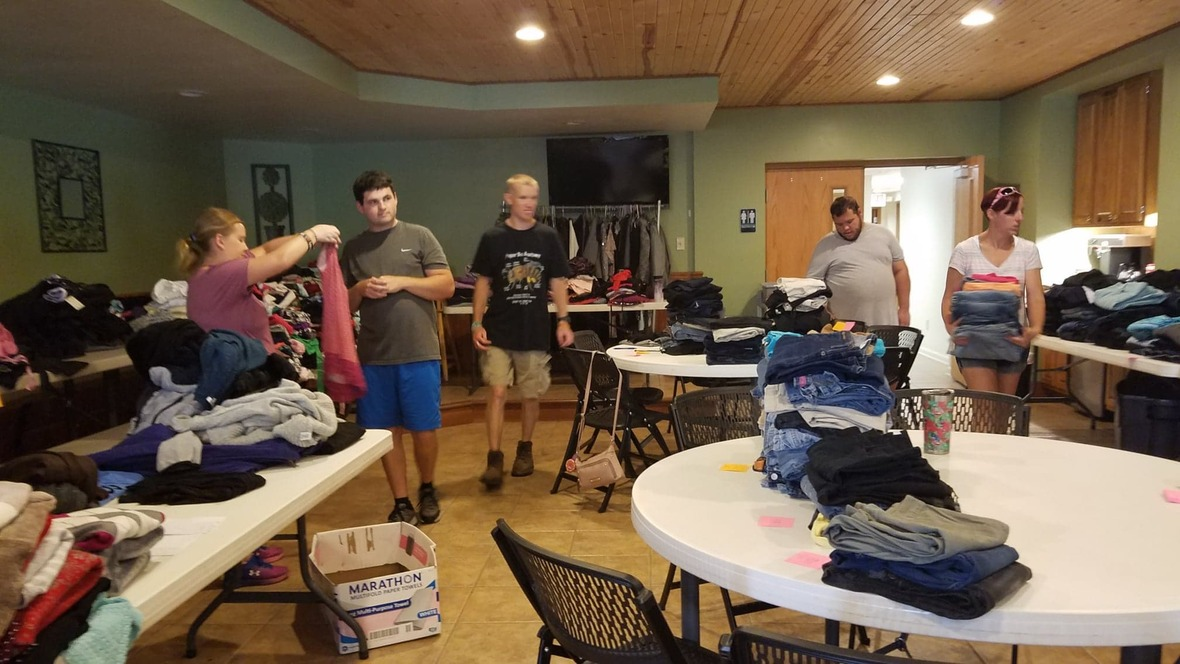/* styles */ ~~~~~~~
 /* styles */ For I was hungry and you gave me food; I was thirsty and you gave me a drink; I was a stranger and you took me in; I was naked and you clothed me; I was sick and you visited me. I was in prison and you came to me. .....When you did it to one of the least of these my brothers and sisters, you were doing it to me. Matthew 25: 35-36, 40 ~ What can YOU do?
 table div table+table+table+table+table+table+table+table+table+table+table+table+table+table+table+table+table+table+table+table+table+table+table+table+table+table+table+table+table+table+table+table+table+table+table+table+table+table+table+table div table{width:100%;padding:0}table div table+table+table+table+table+table+table+table+table+table+table+table+table+table+table+table+table+table+table+table+table+table+table+table+table+table+table+table+table+table+table+table+table+table+table+table+table+table+table+table div table img{width:96.23%;padding:0;float:none}table div table+table+table+table+table+table+table+table+table+table+table+table+table+table+table+table+table+table+table+table+table+table+table+table+table+table+table+table+table+table+table+table+table+table+table+table+table+table+table+table div table td{width:100%;padding:0 1.88% 18px}/* styles */# LOCAL COMMUNITY NEWS/EVENTS

 /* styles */ If you know of any interesting news or events, please let us know, so that we can pass it on to others!

## Local Fall Fun

Hayrides to the Pumpkin Patch at Detwiler Farm
Saturday & Sunday from 11am-5pm
Hayrides are \$6 per person. Age 2 and under ride free. Cost includes a 15-minute hayride to the 10-acre pumpkin patch, an indoor straw maze, a 4-acre cornfield maze and petting zoo. Hayrides leave about every 15 minutes. Pumpkins are weighed and purchased for \$0.37/lb once you return from the pumpkin patch. (Saturdays and Sundays, Sept 28-Oct. 27)

 /* styles */ Mill Creek Park Scarecrow Building at Fellows Riverside Gardens October 5, 9am-12pm Have fun creating your own scarecrow to be displayed at Fellows Riverside Gardens! Bring old clothes to dress your scarecrow, we will provide the rest. Call FRG for more information. Free.
 table.module-44{width:0%;padding:0}table div table+table+table+table+table+table+table+table+table+table+table+table+table+table+table+table+table+table+table+table+table+table+table+table+table+table+table+table+table+table+table+table+table+table+table+table+table+table+table+table+table+table+table+table+table div table{width:0%;float:none;margin-left:auto;margin-right:auto;padding:0}table div table+table+table+table+table+table+table+table+table+table+table+table+table+table+table+table+table+table+table+table+table+table+table+table+table+table+table+table+table+table+table+table+table+table+table+table+table+table+table+table+table+table+table+table+table div table a{border:0 none;text-decoration:none}table div table+table+table+table+table+table+table+table+table+table+table+table+table+table+table+table+table+table+table+table+table+table+table+table+table+table+table+table+table+table+table+table+table+table+table+table+table+table+table+table+table+table+table+table+table div table img{width:100%!important;border:0 none;text-decoration:none}table div table+table+table+table+table+table+table+table+table+table+table+table+table+table+table+table+table+table+table+table+table+table+table+table+table+table+table+table+table+table+table+table+table+table+table+table+table+table+table+table+table+table+table+table+table div table td{width:100%;padding:0}/* styles */
 table.module-45{width:0%;padding:0}table div table+table+table+table+table+table+table+table+table+table+table+table+table+table+table+table+table+table+table+table+table+table+table+table+table+table+table+table+table+table+table+table+table+table+table+table+table+table+table+table+table+table+table+table+table+table div table{width:0%;float:none;margin-left:auto;margin-right:auto;padding:0}table div table+table+table+table+table+table+table+table+table+table+table+table+table+table+table+table+table+table+table+table+table+table+table+table+table+table+table+table+table+table+table+table+table+table+table+table+table+table+table+table+table+table+table+table+table+table div table a{border:0 none;text-decoration:none}table div table+table+table+table+table+table+table+table+table+table+table+table+table+table+table+table+table+table+table+table+table+table+table+table+table+table+table+table+table+table+table+table+table+table+table+table+table+table+table+table+table+table+table+table+table+table div table img{width:100%!important;border:0 none;text-decoration:none}table div table+table+table+table+table+table+table+table+table+table+table+table+table+table+table+table+table+table+table+table+table+table+table+table+table+table+table+table+table+table+table+table+table+table+table+table+table+table+table+table+table+table+table+table+table+table div table td{width:100%;padding:0}/* styles */
 table.module-46{width:0%;padding:0}table div table+table+table+table+table+table+table+table+table+table+table+table+table+table+table+table+table+table+table+table+table+table+table+table+table+table+table+table+table+table+table+table+table+table+table+table+table+table+table+table+table+table+table+table+table+table+table div table{width:0%;float:none;margin-left:auto;margin-right:auto;padding:0}table div table+table+table+table+table+table+table+table+table+table+table+table+table+table+table+table+table+table+table+table+table+table+table+table+table+table+table+table+table+table+table+table+table+table+table+table+table+table+table+table+table+table+table+table+table+table+table div table a{border:0 none;text-decoration:none}table div table+table+table+table+table+table+table+table+table+table+table+table+table+table+table+table+table+table+table+table+table+table+table+table+table+table+table+table+table+table+table+table+table+table+table+table+table+table+table+table+table+table+table+table+table+table+table div table img{width:100%!important;border:0 none;text-decoration:none}table div table+table+table+table+table+table+table+table+table+table+table+table+table+table+table+table+table+table+table+table+table+table+table+table+table+table+table+table+table+table+table+table+table+table+table+table+table+table+table+table+table+table+table+table+table+table+table div table td{width:100%;padding:0}/* styles */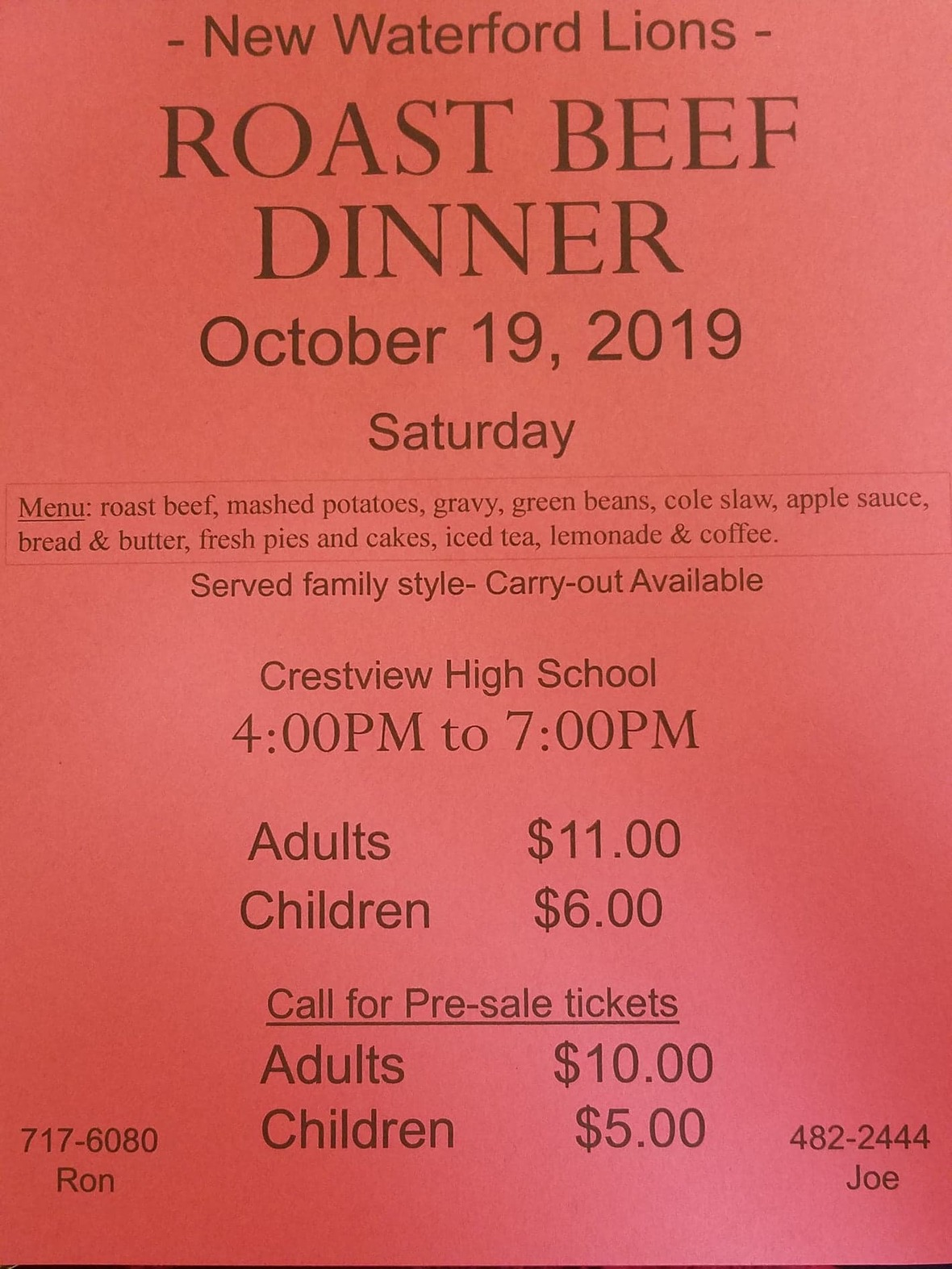/* styles */ See Frank Snyder for tickets.
 table div table+table+table+table+table+table+table+table+table+table+table+table+table+table+table+table+table+table+table+table+table+table+table+table+table+table+table+table+table+table+table+table+table+table+table+table+table+table+table+table+table+table+table+table+table+table+table+table+table+table div table{width:100%;padding:0}table div table+table+table+table+table+table+table+table+table+table+table+table+table+table+table+table+table+table+table+table+table+table+table+table+table+table+table+table+table+table+table+table+table+table+table+table+table+table+table+table+table+table+table+table+table+table+table+table+table+table div table img{width:96.23%;padding:0;float:none}table div table+table+table+table+table+table+table+table+table+table+table+table+table+table+table+table+table+table+table+table+table+table+table+table+table+table+table+table+table+table+table+table+table+table+table+table+table+table+table+table+table+table+table+table+table+table+table+table+table+table div table td{width:100%;padding:0 1.88% 18px}/* styles */# CELEBRATE!

October 4 - Townsend & Sarah Bendersmith
October 4 - World Animal Day
October 5 - National Do Something Nice Day
October 5 - World Teachers' Day (Have you thanked a teacher lately?)
October 6 - Bob & Lynn Yoder
October 6 - Paxton Bachelder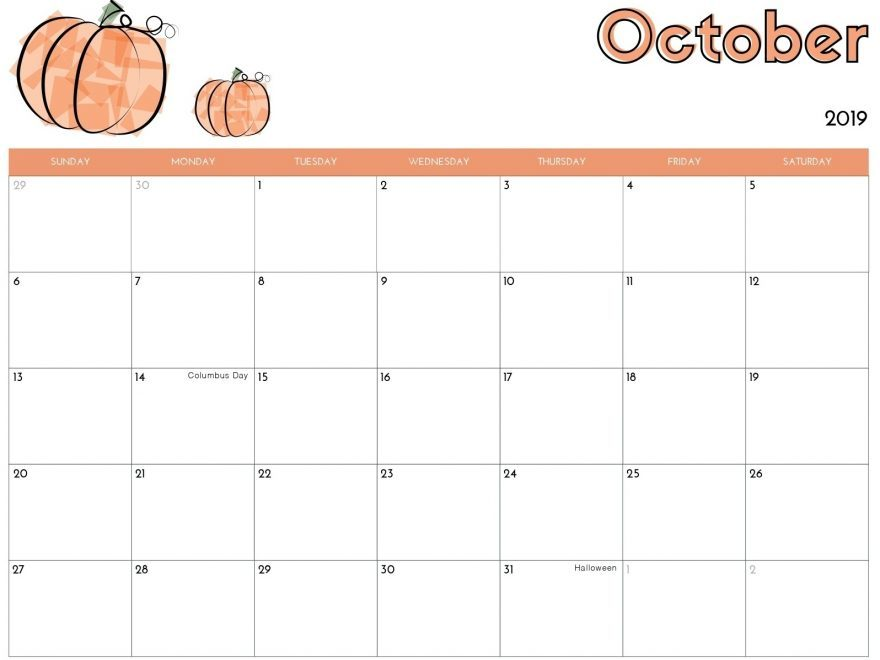# ON THE CG CALENDAR

October 3-9 . . .
Today - Transition Class
Today, 4-7pm - CSA Pick-up
Sunday, 9:30am - Bible Study
Sunday, 11am - Worship
Sunday, 6pm - YG
Monday, 9:30am - GG at Whispering Pines
Tuesday - Leetonia Class
Wednesday, 9:30am - Ladies Bible Study
Wednesday - Mohawk Class/* styles */ Seeking to reflect the unconditional love of God, our mission is to help people connect - to God, to one another, and to God's purpose for the world.
 table div table+table+table+table+table+table+table+table+table+table+table+table+table+table+table+table+table+table+table+table+table+table+table+table+table+table+table+table+table+table+table+table+table+table+table+table+table+table+table+table+table+table+table+table+table+table+table+table+table+table+table+table+table+table+table+table div table{width:100%;padding:0}table div table+table+table+table+table+table+table+table+table+table+table+table+table+table+table+table+table+table+table+table+table+table+table+table+table+table+table+table+table+table+table+table+table+table+table+table+table+table+table+table+table+table+table+table+table+table+table+table+table+table+table+table+table+table+table+table div table img{width:96.23%;padding:0;float:none}table div table+table+table+table+table+table+table+table+table+table+table+table+table+table+table+table+table+table+table+table+table+table+table+table+table+table+table+table+table+table+table+table+table+table+table+table+table+table+table+table+table+table+table+table+table+table+table+table+table+table+table+table+table+table+table+table div table td{width:100%;padding:0 1.88% 18px}/* styles *//* styles */ Come grow with us! Service Time: Sunday @ 11:00am Common Ground Church Community 2310 West South Range Road North Lima, Ohio 44452 ~~~~~~~~~~~~~~~~~~~~~~~~~~~~~~~~~~~~~~~~~~~~ For more information, call 330-549-9408 or contact us by email at commonground.secretary@gmail.com.
 /* styles */